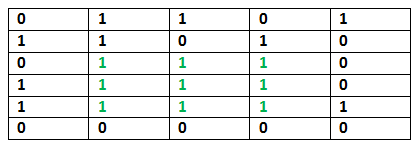# C Program for Maximum size square sub-matrix with all 1s

• Last Updated : 12 Dec, 2018

Given a binary matrix, find out the maximum size square sub-matrix with all 1s.

For example, consider the below binary matrix.## C/C++

 `// C/C++ code for Maximum size square``// sub-matrix with all 1s``#include ``#define bool int``#define R 6``#define C 5`` ` `/* UTILITY FUNCTIONS */``/* Function to get minimum of three values */``int` `min(``int` `a, ``int` `b, ``int` `c)``{``    ``int` `m = a;``    ``if` `(m > b)``        ``m = b;``    ``if` `(m > c)``        ``m = c;``    ``return` `m;``}`` ` `void` `printMaxSubSquare(``bool` `M[R][C])``{``    ``int` `i, j;``    ``int` `S[R][C];``    ``int` `max_of_s, max_i, max_j;`` ` `    ``/* Set first column of S[][]*/``    ``for` `(i = 0; i < R; i++)``        ``S[i] = M[i];`` ` `    ``/* Set first row of S[][]*/``    ``for` `(j = 0; j < C; j++)``        ``S[j] = M[j];`` ` `    ``/* Construct other entries of S[][]*/``    ``for` `(i = 1; i < R; i++) {``        ``for` `(j = 1; j < C; j++) {``            ``if` `(M[i][j] == 1)``                ``S[i][j] = min(S[i][j - 1], S[i - 1][j],``                              ``S[i - 1][j - 1])``                          ``+ 1;``            ``else``                ``S[i][j] = 0;``        ``}``    ``}`` ` `    ``/* Find the maximum entry, and indexes of maximum entry ``    ``in S[][] */``    ``max_of_s = S;``    ``max_i = 0;``    ``max_j = 0;``    ``for` `(i = 0; i < R; i++) {``        ``for` `(j = 0; j < C; j++) {``            ``if` `(max_of_s < S[i][j]) {``                ``max_of_s = S[i][j];``                ``max_i = i;``                ``max_j = j;``            ``}``        ``}``    ``}`` ` `    ``printf``(``"Maximum size sub-matrix is: \n"``);``    ``for` `(i = max_i; i > max_i - max_of_s; i--) {``        ``for` `(j = max_j; j > max_j - max_of_s; j--) {``            ``printf``(``"%d "``, M[i][j]);``        ``}``        ``printf``(``"\n"``);``    ``}``}`` ` ` ` ` ` `/* Driver function to test above functions */``int` `main()``{``    ``bool` `M[R][C] = { { 0, 1, 1, 0, 1 },``                     ``{ 1, 1, 0, 1, 0 },``                     ``{ 0, 1, 1, 1, 0 },``                     ``{ 1, 1, 1, 1, 0 },``                     ``{ 1, 1, 1, 1, 1 },``                     ``{ 0, 0, 0, 0, 0 } };`` ` `    ``printMaxSubSquare(M);``    ``getchar``();``}`

Output:

```Maximum size sub-matrix is:
1 1 1
1 1 1
1 1 1
```

Please refer complete article on Maximum size square sub-matrix with all 1s for more details!

My Personal Notes arrow_drop_up